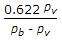# Mechanical Engineering - Heat Transfer, Refrigeration and Air Conditioning

### Exercise :: Heat Transfer, Refrigeration and Air Conditioning - Section 6

21.

The expressionis used for calculating

 A. relative humidity B. humidity ratio C. degree of saturation D. pressure of water vapour

Explanation:

No answer description available for this question. Let us discuss.

22.

The critical thickness of insulation for a sphere is

 A. k/h0 B. 2k/h0 C. h0/k D. h0/2k

Explanation:

No answer description available for this question. Let us discuss.

23.

The difference between dry bulb temperature and dew point temperature, is called

 A. dry bulb depression B. wet bulb depression C. dew point depression D. degree of saturation

Explanation:

No answer description available for this question. Let us discuss.

24.

Logarithmic mean temperature difference is the same as the arithmetic mean temperature difference.

 A. Agree B. Disagree

Explanation:

No answer description available for this question. Let us discuss.

25.

Film coefficient is defined as the ratio of

 A. thermal conductivity to the equivalent thickness of the film of fluid B. temperature drop through the films of fluids to the thickness of film of fluids C. thickness of film of fluid to the thermal conductivity D. thickness of film of fluid to the temperature drop through the films of fluids